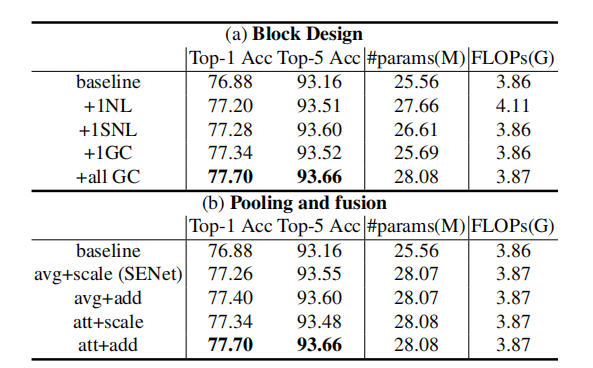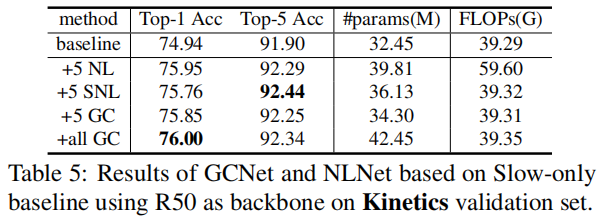# 【CV中的Attention机制】Non-Local改进CCNet¶

GCNet论文名称为：《GCNet: Non-local Networks Meet Squeeze-Excitation Networks and Beyond》，是由清华大学提出的一个注意力模型，与SE block、Non Local block类似，提出了GC block。为了克服NL block计算量过大的缺点，提出了一个Simplified NL block，由于其与SE block结构的相似性，于是在其基础上结合SE改进得到GC block。

SENet中提出的SE block是使用全局上下文对不同通道进行权值重标定，对通道依赖进行调整。但是采用这种方法，并没有充分利用全局上下文信息。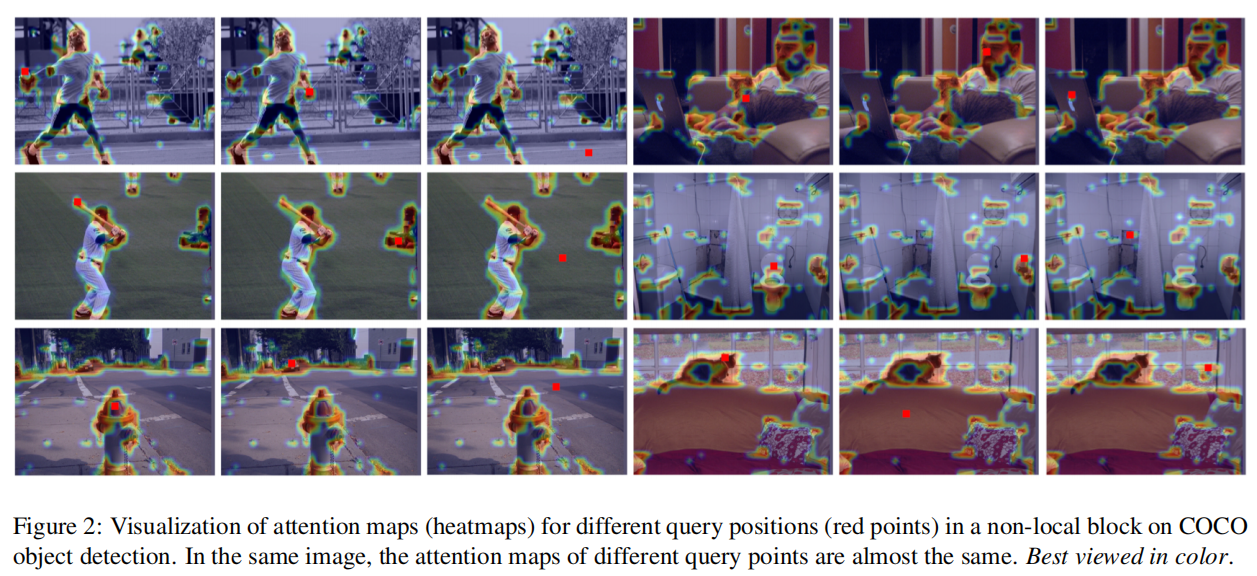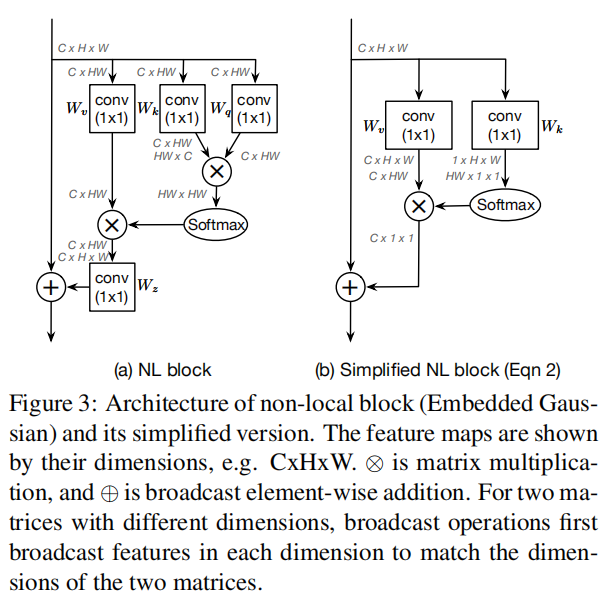NLNet 中的Non-Local block可以表示为：

z_i=x_i+W_z\sum^{N_p}_{j=1}\frac{f(x_i,x_j)}{C(x)}(W_v×x_j)

NLNet中提出了四个相似度计算模型，其效果是大概相似的。作者以Embedded Gaussian为基础进行改进，可以表达为:

W_{ij}=\frac{exp(W_qx_i,W_kx_j)}{\sum_{m}exp(W_qx_i,W_kx_m)}

z_i=x_i+W_v\sum^{N_p}_{j=1}\frac{exp(W_kx_j)}{\sum^{N_p}_{m=1}exp(W_kx_m)}x_jGC block在ResNet中的使用位置是每两个Stage之间的连接部分，下边是GC block的官方实现(基于mmdetection进行修改)：

import torch
from torch import nn

class ContextBlock(nn.Module):
def __init__(self,inplanes,ratio,pooling_type='att',
super(ContextBlock, self).__init__()

assert pooling_type in ['avg', 'att']
assert isinstance(fusion_types, (list, tuple))
assert all([f in valid_fusion_types for f in fusion_types])
assert len(fusion_types) > 0, 'at least one fusion should be used'

self.inplanes = inplanes
self.ratio = ratio
self.planes = int(inplanes * ratio)
self.pooling_type = pooling_type
self.fusion_types = fusion_types

if pooling_type == 'att':
self.softmax = nn.Softmax(dim=2)
else:
nn.Conv2d(self.inplanes, self.planes, kernel_size=1),
nn.LayerNorm([self.planes, 1, 1]),
nn.ReLU(inplace=True),  # yapf: disable
nn.Conv2d(self.planes, self.inplanes, kernel_size=1))
else:
if 'channel_mul' in fusion_types:
self.channel_mul_conv = nn.Sequential(
nn.Conv2d(self.inplanes, self.planes, kernel_size=1),
nn.LayerNorm([self.planes, 1, 1]),
nn.ReLU(inplace=True),  # yapf: disable
nn.Conv2d(self.planes, self.inplanes, kernel_size=1))
else:
self.channel_mul_conv = None

def spatial_pool(self, x):
batch, channel, height, width = x.size()
if self.pooling_type == 'att':
input_x = x
# [N, C, H * W]
input_x = input_x.view(batch, channel, height * width)
# [N, 1, C, H * W]
input_x = input_x.unsqueeze(1)
# [N, 1, H, W]
# [N, 1, H * W]
# [N, 1, H * W]
# [N, 1, H * W, 1]
# [N, 1, C, 1]
# [N, C, 1, 1]
context = context.view(batch, channel, 1, 1)
else:
# [N, C, 1, 1]
context = self.avg_pool(x)
return context

def forward(self, x):
# [N, C, 1, 1]
context = self.spatial_pool(x)
out = x
if self.channel_mul_conv is not None:
# [N, C, 1, 1]
channel_mul_term = torch.sigmoid(self.channel_mul_conv(context))
out = out * channel_mul_term
# [N, C, 1, 1]
return out

if __name__ == "__main__":
in_tensor = torch.ones((12, 64, 128, 128))

cb = ContextBlock(inplanes=64, ratio=1./16.,pooling_type='att')

out_tensor = cb(in_tensor)

print(in_tensor.shape)
print(out_tensor.shape)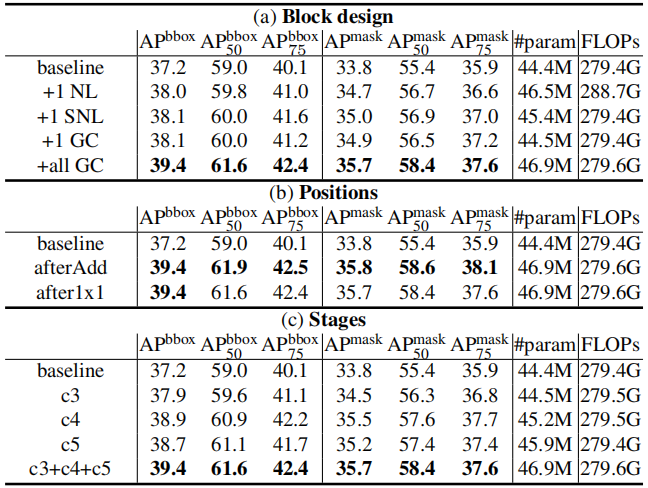• 从block设计来讲，可以看出Simplified NL与NL几乎一直，但是参数量要小一些。而每个阶段都使用GC block的情况下能比baseline提高2-3%。
• 从添加的不同阶段来看，施加在三个阶段效果最优，能比baseline高1-3%。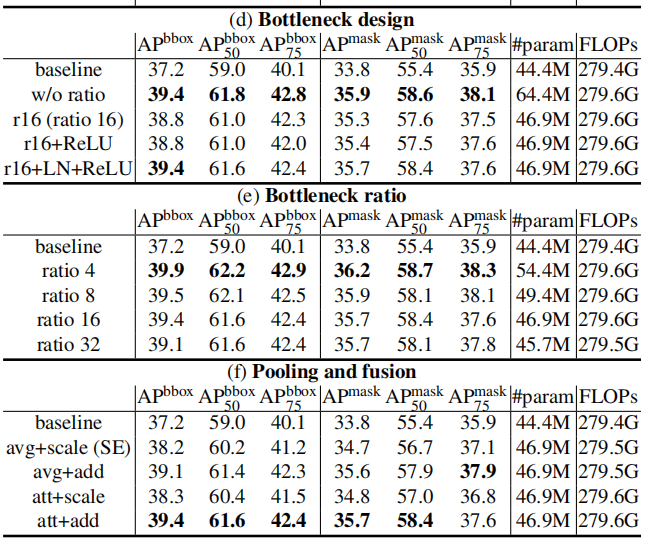• Bottleneck设计方面，测试使用缩放、ReLU、LayerNorm等组合，发现使用简化版的NLNet并使用1×1卷积作为transform的时候效果最好，但是其计算量太大。

• 缩放因子设计：发现ratio=¼的时候效果最好。